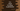# C++ program to check if a string is palindrome or not## Introduction :

A palindrome string is a string that is equal from both sides. For example, madam, radar, level, mom are palindrome strings. In this post, we will learn how to find if a string is palindrome or not in C++.

We will write one C++ program to take one string from the user as input. It will ask the user to enter the string. It will read that string and pass it to a different function to check if it is palindrome or not. If it is a palindrome, it will print out that the string is a palindrome. Else, it will print out that the string is not a palindrome.

### C++ program to check palindrome method 1: Using a loop :

``````#include <iostream>
using namespace std;

bool isPalindrome(string str)
{
int j = str.length() - 1;

for (int i = 0; i < j; i++, j--)
{
if (str[i] != str[j])
{
return false;
}
}

return true;
}

int main()
{
string words = {"mom", "radar", "level", "hello", "one"};
for (int i = 0; i < 5; i++)
{
if (isPalindrome(words[i]))
{
cout << words[i] << " -> Palindrome" << endl;
}
else
{
cout << words[i] << " -> Not a Palindrome" << endl;
}
}

return 0;
}``````

### Explanation :

1. In this program, isPalindrome method checks if a string is a palindrome or not. It takes one string and compares each letter with the direct opposite letter.
2. words is a string array. We have five strings and we are checking for each string if it is palindrome or not.
3. We are printing one message based on if the string is palindrome or not.

### Output :

This program will print the below output :

``````mom -> Palindrome
level -> Palindrome
hello -> Not a Palindrome
one -> Not a Palindrome``````

### C++ program to check palindrome method 2: By reversing the string :

We can also reverse the string and compare it with the original string to find out if the string is palindrome or not. The below program does that :

``````#include <iostream>
#include <string.h>
using namespace std;

bool isPalindrome(string str)
{
string newString = str;
reverse(newString.begin(), newString.end());
return str.compare(newString) == 0;
}

int main()
{
string words = {"mom", "radar", "level", "hello", "one"};
for (int i = 0; i < 5; i++)
{
if (isPalindrome(words[i]))
{
cout << words[i] << " -> Palindrome" << endl;
}
else
{
cout << words[i] << " -> Not a Palindrome" << endl;
}
}

return 0;
}``````

### Explanation :

We are using the same array of strings as the above example. isPalindrome method reverses the string using the reverse method and compares it with the old string using str.compare. If the return value of this method is 0, it means that both strings are equal i.e. the provided string is a palindrome.

The output is the same as the previous example.